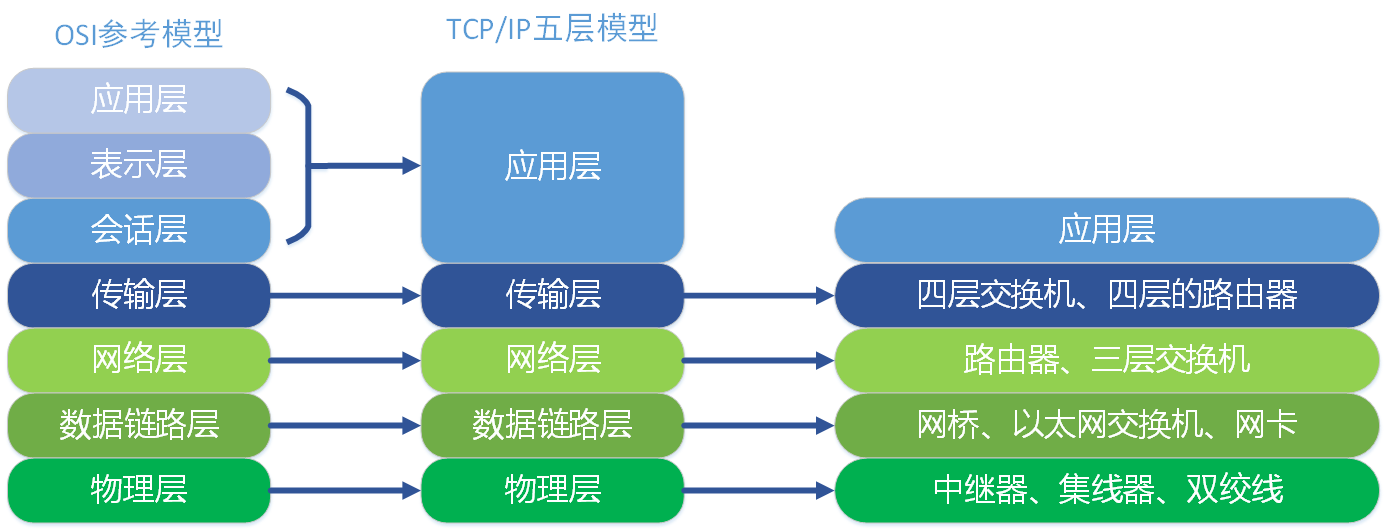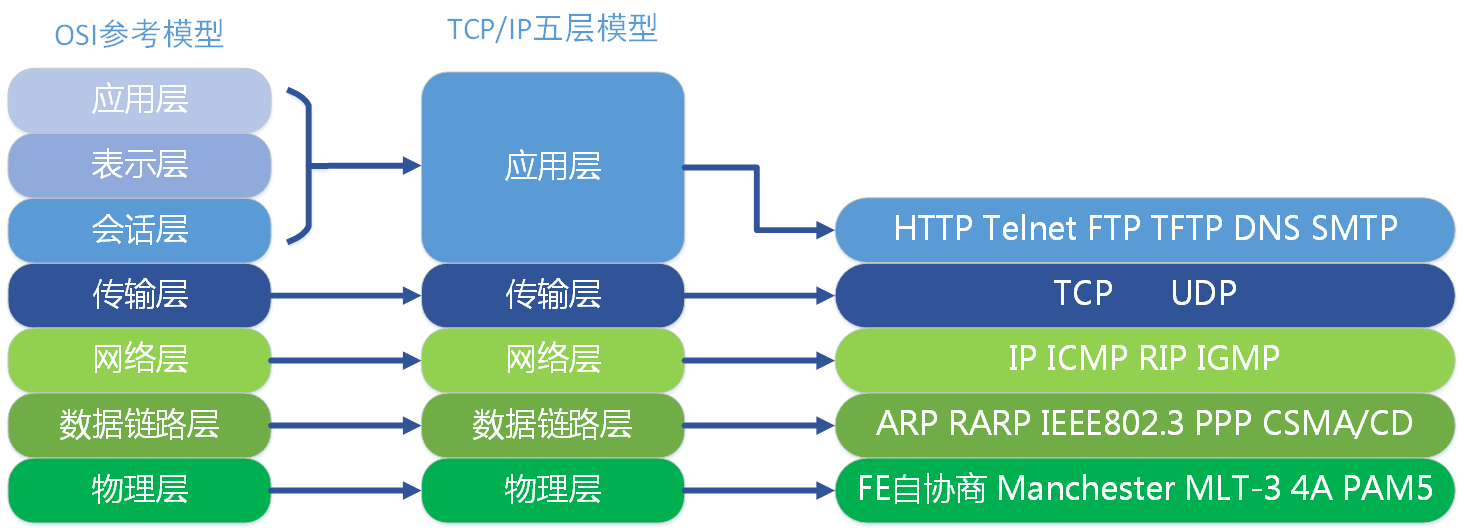# 初赛基础

By 缪慎浩

## 计算机语言

• ### 机器语言

我们知道，计算机内部是以二进制存储数据的。同理，对于指令自然也是以二进制存储的。

所以我们就可以直接用二进制代码来写程序，比如“计算8+4”就可以写为“00001000 00000100 00000100”。但我们要知道不同CPU的指令集是不一样的

显然地，这玩意儿很反人类，但这是计算机可以直接识别直接运行的语言，故我们称之为“机器语言”。

• ### 汇编语言

由于二进制代码直接编程十分*蛋，聪明的人类就利用一些助记符，比如一些单词 “mov” 之类的来代替二进制码然后编程，这让编程轻松了很多，不过汇编程序必须经过翻译后才能运行。

然鹅由于汇编语言并没有做出本质上的改变，只是给二进制命令改了个名，我们仍将其称为低级语言。其具有编写逻辑困难并十分反智等特点，而且由于不同CPU指令集不同，所以汇编程序的可移植性也奇差。

汇编程序速度并不一定是最快的。程序的运行速度只取决于最后的指令条数以及CPU执行速度。

### 高级语言

• 编译型语言：C/C++ Basic
• 解释型语言：PHP Python Swift Java Matlab

## 数制转换

例子： 11001001B = 105D = 311O = D9H


### 二进制$\leftrightarrows$八进制$\leftrightarrows$十六进制

• O to B 把八进制一位换成二进制三位即可。（看举例）
• B to O 每三位转成8进制即可
• H to B 把每一位转换成二进制四位即可。
• B to H 每四位按权展开即可。

### 十进制$\leftrightarrows$二进制

• D to B 整数部分转为二进制整数可用“除二求余，逆序输出”的方法。
• D to B 小数部分转为二进制小数可用“乘二取整，顺序输出”的方法。（看黑板举例）
• B to D 直接按权值展开
• 注意：二进制无限循环小数可能是个十进制的有限小数，但是十进制无限循环小数，一定不会是个二进制有限小数

### 课堂练兵 迅速口答

• $5D + 19H = ? B$
• $15O + 10010B = ? D$
• $98AH + 43D = ? O$
• $43D + 32O = ? H$
• $4O + 14H = ? B$

## 数在计算机中的表示

• 整数表示
• 实数表示

### 整数表示：原码，补码，反码

• 有原码，补码，反码三种方式

• 有如下公式：转换时仅比 $x$ 增加一位，即符号位。字符串的加号是连接符号。

### 实数表示：定点数，浮点数

• 整数就是一种十分常见的定点数，它是一种小数点固定在最后一位之后的数。
• 因为定点数表示小数的精度明显不够，浮点数应运而生。这是一种小数点位置不固定的数，它由一个定点数，乘一个基数的幂次得到，所以浮点数被分为尾数和阶码，尾数表示有效数值，而阶码表示小数点位置，定义 $E$ 为阶码，$s$ 为符号，$M$ 为尾数，得到计算公式 $V = (-1)^s * M * 2^E$ 这与人类采用的十进制科学计数法十分相似 $114514 = 1.14514 * 10^5$ 这样我们可以固定小数点的位置。
IEEE 754 浮点数位数E 的长度M 的长度

• 局域网（LAN

• 城域网（MAN）

• 广域网（WAN）

• 星形
• 总线形
• 环形
• 树形
• 网状形

##